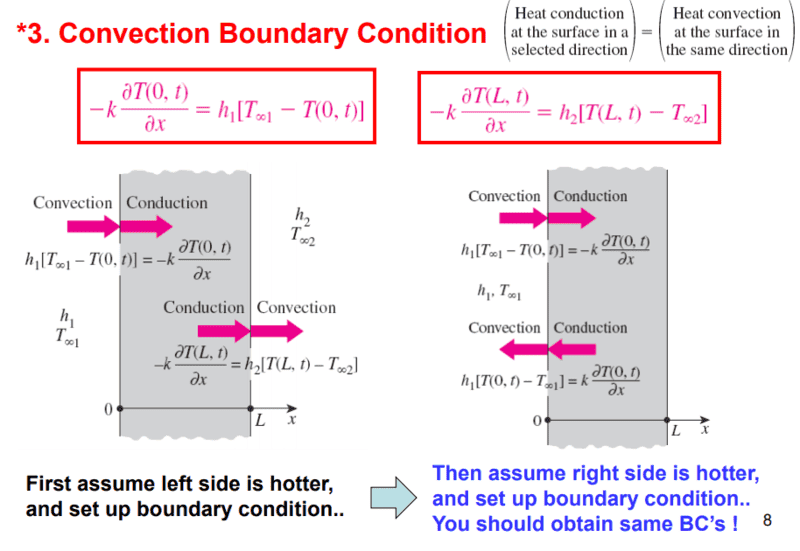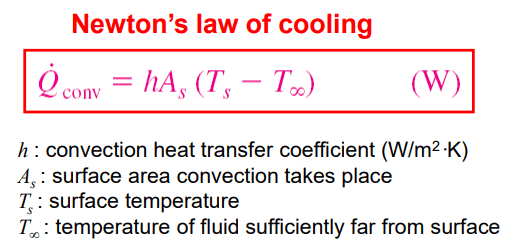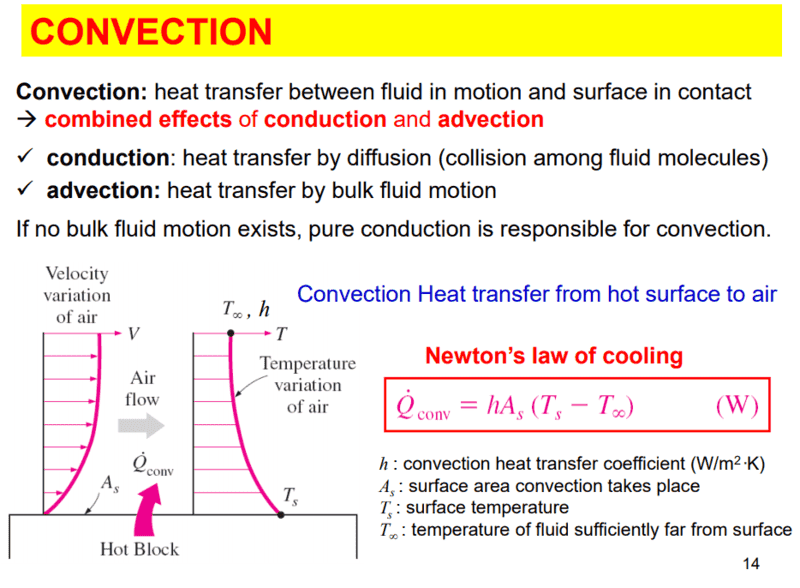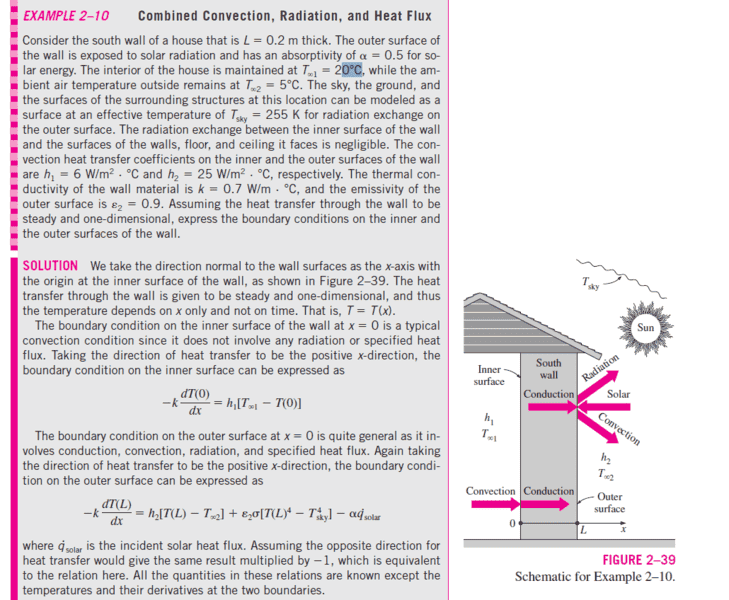# Heat transfer boundary condition

• EastWindBreaks

## Homework StatementI am confused on how it's using the surrounding temperature minus the surface temperature as its the other way around in the Newton's law of cooling, Doing that would change the sign of convection right? I don't see the reason to do that, since if left side is hotter, then conduction is positive going from left to right.

## Homework Equations## The Attempt at a Solution

#### Attachments

What is the context for the form of Newton's Law that you quote? Seems to me that T here refers to the ambient temperature in the heat sink. In the image you post, T∞1 is the distal temperature in the heat source.

What is the context for the form of Newton's Law that you quote? Seems to me that T here refers to the ambient temperature in the heat sink. In the image you post, T∞1 is the distal temperature in the heat source.
it's from here :isn't distal temperature same as ambient temperature in this case? so I guess if surface is cooling, then we have to take surface temperature minus ambient temperature, if the surface is being heated, then we have to take the ambient temperature minus surface temperature? but sometimes even when the surface temperature is not given, the book still uses" ambient temperature minus surface temperature" on the left side of the wall. like this example from the book:#### Attachments

isn't distal temperature same as ambient temperature in this case?
Yes, but as I posted, one equation is looking at the ambient temperature in the heat source, while the other, Newton, is looking at the ambient temperature in the heat sink.

•EastWindBreaks
Your book isn't doing a very good job of explaining this. It has led you think in terms of which temperature is higher in a particular problem and which temperature is lower. This is not the proper way of analyzing this. You need to focus rather on which temperature is situated at lower x, and which temperature is situated at higher x. This is because the heat flux is really a vector quantity. In reality, it should be accompanied by a unit vector in the x direction. If the ambient temperature is at lower x and the wall is at higher x (like say adjacent to the wall at x = 0), then the heat flux vector is $$\mathbf{q}=h(T_{ambient}-T_{wall})\mathbf{i_x}\tag{1}$$It doesn't matter which temperature is higher and which temperature is lower. The temperature at smaller x comes first and the temperature at higher x comes last. Similarly, at the boundary x = L, the wall temperature is situated at lower x than then ambient. Therefore, at that boundary, $$\mathbf{q}=h(T_{wall}-T_{ambient})\mathbf{i_x}\tag{2}$$It doesn't matter which temperature is higher and which temperature is lower.

In Eqn. 1, if the wall temperature is higher than the ambient temperature, all that means is the the heat flux is in the negative x direction (rather than the positive x direction).

•EastWindBreaks
Your book isn't doing a very good job of explaining this. It has led you think in terms of which temperature is higher in a particular problem and which temperature is lower. This is not the proper way of analyzing this. You need to focus rather on which temperature is situated at lower x, and which temperature is situated at higher x. This is because the heat flux is really a vector quantity. In reality, it should be accompanied by a unit vector in the x direction. If the ambient temperature is at lower x and the wall is at higher x (like say adjacent to the wall at x = 0), then the heat flux vector is $$\mathbf{q}=h(T_{ambient}-T_{wall})\mathbf{i_x}\tag{1}$$It doesn't matter which temperature is higher and which temperature is lower. The temperature at smaller x comes first and the temperature at higher x comes last. Similarly, at the boundary x = L, the wall temperature is situated at lower x than then ambient. Therefore, at that boundary, $$\mathbf{q}=h(T_{wall}-T_{ambient})\mathbf{i_x}\tag{2}$$It doesn't matter which temperature is higher and which temperature is lower.

In Eqn. 1, if the wall temperature is higher than the ambient temperature, all that means is the the heat flux is in the negative x direction (rather than the positive x direction).
wow, you are right, I got stuck on trying to write that term base on which temperature is higher and which is lower, thank you very much for the awesome explanation!